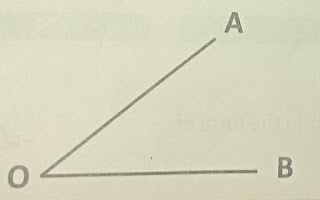# Lesson Planning of Angles

Lesson Planning of Angle and its Recognition

Subject Mathematics

Students` Learning Outcomes

• Recognize an angle through non-parallel lines.
• Draw and angle AOB with vertex (O) and arrow OA and OB
• Recognize notation AOB

Information for Teachers

• A line has no beginning point or end point. Imagine it continuing infinity to both directions. We can illustrate that by little arrows on both ends.
• A line segment has a beginning point and an end point or line segment has two end points.
• A ray has a beginning point but no end point or ray has one end point. Think of sun`s rays; they start at sun and go on forever.

• What is an angle? In mathematics, an angle is made up of two rays that have the same beginning arms point. That point is called the vertex and the two rays are called the sides (arms) of the angle.
• While writing an angle we put the vertex name always at the middle and the symbol of angle is written “• During this lesson, teacher should also consult textbook wherein and whenever it is required.

Material / Resources

Writing board, chalk/marker, duster, geometry box, two pencils, pebbles, textbook

Introduction

 Worm up Activity 1 Do you know the word “ankle”, the bend between the leg and the foot? With this ankle you can bend your foot in semi-circular way. Do you know where your elbow is? You can bend your arm because of it.Ask students to take out their divider from geometry box and see what makes the two arms bend? Introduce center point as “vertex” and sides.

 Worm up Activity 2 Take two pencils and place them together and rotate one of them slowly and demonstrate the making of angle as shown in figure 2. Ask students to take any two pencils and rotate the same way and identify vertex and sides. Ask students to look for bends or angles in their surroundings.Development

 Activity 1 On board draw two lines in v shape and label vertex, sides and angles. Label it with O, A and B and give your input about labeling it.Repeat the concept as many times so that they understand. Draw few figures on board and label it with different alphabets and ask students to name the angles like ∠AOB Give them 5 minutes to think and then invite individuals to label it on board.

Sum up / Conclusion

• Line, ray and line segment has two, one and no end point respectively.
• An angle is made up of two rays that have the same beginning arms point, that point is called the vertex.• The angle between OA and OB is denoted by AOB or BOA.

Assessment

• Questions can be given to students in the form of worksheet or by writing on board
1. How many vertexes are there in this figure?
2. How many rays are there in this figure?
3. What is the common point of the two rays?
4. Which point is taken at the middle while writing the name of an angle?
5. Name the angle.